### RSLColor By Height

#### Introduction

This tutorial addresses some issues relating to the shading of surfaces based on their position in world-space, in particular, their height above the origin of the world coordinate system. For example, a shader may be required to tint the leaves of a tree based on their height above the ground. A model of a tree might consist of several hundreds of leaves, consequently, it would be beneficial to have a single instance of a shader control the shading of the entire canopy of leaves.

##### Modeling & Coloration

Since colors can be specified in rib file (or rib stream) why not assign a color to each leaf when it is modeled? For example, the snippet of rib shown below assigns a color to a polygon that represents a single leaf.

```    AttributeBegin
Attribute "identifier" "string name" ["leaf45"]
Translate 0 2 0
Color 0.772 0.964 0.772...
Polygon ...
AttributeEnd```

Typically, a model of a canopy of leaves would be baked as a rib archive. A shader assigned to the canopy would have access to the color of each leaf via the `Cs` primitive variable. However, the problem with assigning colors in this way is that changes to the coloration must be done by regenerating the rib file or by post-processing the pre-baked rib file with a Rif filter. An alternative approach is to use a helper app (listings 5 & 6) to generate a rib stream that specifies the geometry at render-time ie. a procedural primitive. Refer to the tutorial "RenderMan Procedural Primitives'" for information about helper apps.

A more flexible approach is to postpone all decisions about coloration until a scene is shaded. Figures 1 and 2 show three polygons distributed along the x-axis of world-space. In figure 1 the local origin of each polygon is marked by small black spheres.Figure 1 Correct uniform shading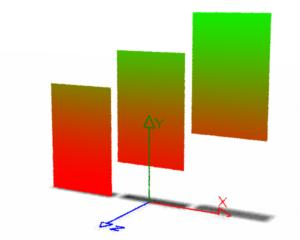Figure 2 Vertical color blending, refer to shader listing 1

 In general it is assumed the shaders developed in this tutorial should apply a constant colorization to the surface(s) to which they are assigned - figure 1. Applying coloration based on the height of the micro-polygon being shaded will produce vertical color blending that, under some circumstances, may be undesireable - figure 2. However, as shown in figures 3a and 3b, when the polygons representing the leaves are small the effect of vertically blended coloration caused by taking the height of `P` is less noticeable. As will be shown later, when the polygons are moving up and down other issues come into play.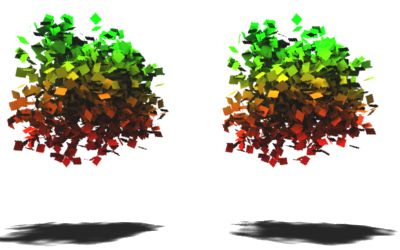Figures 3a and 3b
 Left: coloration by the height of the polygon origin. Right: coloration by the height of each micro-polygon (P).

#### Coloration by Micro-Polygon Height

Listing 1 demonstrates a method of assigning a color to `Ci` based on the height of each micro-polygon. The code creates a copy of `P` transformed to "world" space, the 'y' component of which is obtained by direct indexing,
`p`
alternately, the RSL function,
`ycomp(p)`
could be used to access the 'y' value.

Listing 1 - colorByP.sl

 ```surface colorByP(float Kfb = 1, minheight = 1.25, maxheight = 2.5; color mincolor = color(1,0,0), maxcolor = color(0,1,0)) { point p = transform("world", P); float blend = smoothstep(minheight, maxheight, p); color surfcolor = mix(mincolor, maxcolor, blend); Oi = Os; Ci = Oi * surfcolor * Kfb; }```

For convenience, the shader uses the `smoothstep()` function to obtain a normalized value suitable for mixing colors. However, a linear blending could be used, for example,

```    //float    blend = smoothstep(minheight, maxheight, p);
float    blend = 1;
if(p > minheight && p < maxheight)
blend = (p - minheight) / (maxheight - minheight);
else if(p <= minheight)
blend = 0;```

For simplicity the shader does not perform any lighting calculations.

#### Coloration by Surface Origin

Listing 2 demonstrates a method of obtaining the height of the local origin of a surface above the origin of the world coordinate system. A modified version of this shader was used to render figure 3a.

Listing 2 - colorByObjOrigin.sl

 ```surface colorByObjOrigin(float Kfb = 1, minheight = 1.25, maxheight = 2.5; color mincolor = color(1,0,0), maxcolor = color(0,1,0)) { // The xyz values of "originC" will not be 0,0,0 // because they will be (automatically) converted to // current space aka. the "camera" coordinate system. point originC = point "object" (0,0,0); // Because of the use of the transform() function // the xyz values of "originW" will not be converted to // "camera" space but will retain their true "world" // space values. point originW = transform("world", originC); float height = originW; float blend = smoothstep(minheight, maxheight, height); color surfcolor = mix(mincolor, maxcolor, blend); Oi = Os; Ci = Oi * surfcolor * Kfb; } ```

#### Coloration by User Attribute

Listing 3 demonstrates a method of coloring a surface based on a user attribute called "position". The values of the attribute represent either the initial or the current (animated) position of a surface. Basing the coloration of a surface on its initial position will ensure its color does not change during an animation - figure 5.

```    TransformBegin
Attribute "identifier" "string name" ["leaf90"]
Attribute "user" "point position" [0.074 -0.130 0.218]
Transform [....]
Polygon ...
TransformEnd```

Listing 3 - colorByAttribute.sl

 ```surface colorByAttribute(float Kfb = 1, minheight = 1.25, maxheight = 2.5, offset = 1; color mincolor = color(1,0,0), maxcolor = color(0,1,0)) { color surfcolor = Cs; point pos; if(attribute("user:position", pos) == 1) { float ht = offset + pos; float blend = smoothstep(minheight, maxheight, ht); surfcolor = mix(mincolor, maxcolor, blend); } Oi = Os; Ci = Oi * surfcolor * Kfb; }```

#### Animation & Other Issues

Of the three shading techniques presented in this tutorial only the `colorByAttribute` shader is able to retain the color of a surface while its height changes during an animation - figure 5. However, this is true only if the values of the user attributes assigned to the surface are those relating to its initial position.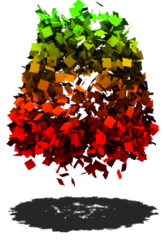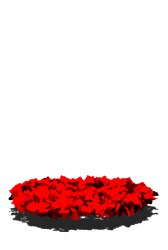Figure 4
 Rendered with the colorByP shader. The same effect is observed when the colorByObjOrigin is used.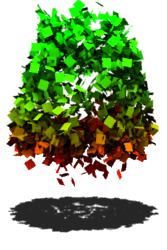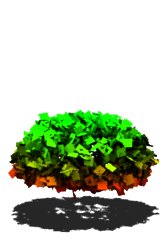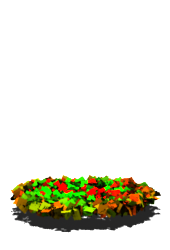Figure 5 Rendered with the colorByAttribute shader

 Although the `colorByObjOrigin` shader works well for collections of static surfaces, care must be taken NOT to apply their transformation matrix to their vertices. In Maya this is done using Modify->Freeze Transformations menu. Doing so will force the local ("object") coordinate system of each surface to coincide with the "world" coordinate system, thus removing the opportunity of the shader to determine the height of a surface above the origin of the "world" space! For more information about this topic refer to the tutorial "Maya:Freeze Transforms".

#### Using a Procedural Primitive to Generate Polygons

The polygon models used in this tutorial were generated as procedural primitives using the (helper app) python script shown in listing 4. For information about helper apps refer to the tutorial "RenderMan Procedural Primitives". A sample rib file that makes use of the helper app is shown in listing 5. If the reader is using the Cutter text editor the `canopy.py` script should be saved in the same directory as the cutter.jar file.

Another python script, named `pnoise.py`, must also be saved in the cutter directory. That script can be found at "Noise:Perlin Improved Noise"

Listing 4 - canopy.py

 ```import sys, math, random from pnoise import pnoise def length(x,y,z): return math.sqrt(x*x + y*y + z*z) def normalize(x,y,z): len = length(x,y,z) return x/len, y/len, z/len def scaleVector(x,y,z,sc): return x * sc,y * sc, z * sc def placePoly(id, x,y,z,ang): result = 'TransformBegin\n' result += ' Attribute "identifier" "string name" ["leaf%d"]\n' % (id) result += ' Attribute "user" "float angle" [%1.3f] \n' % (ang) result += ' Attribute "user" "point position" [%1.3f %1.3f %1.3f]\n' % (x,y,z) result += ' Translate %1.3f %1.3f %1.3f\n' % (x,y,z) rXRot = random.random() rYRot = random.random() rZRot = random.random() result += ' Rotate %1.3f %1.3f %1.3f %1.3f\n' % (ang,rXRot,rYRot,rZRot) result += ' Polygon "P" [-0.0625 0 -0.125 0.0625 0 -0.125 0.0625 0 0 -0.0625 0 0]\n' result += ' "st" [0 0 0 1 1 1 1 0]\n' result += 'TransformEnd\n' return result def main(): args = sys.stdin.readline() while args: random.seed(1) values = args.split() pixels = float(values) numLeaves = float(values) amplitude = float(values) # The polys are randomly distributed within two concentric # noisey spheres. The outer radius is arbitarily set to 1 # unit with the inner radius 20% smaller outerRad = 1.0 innerRad = outerRad * 0.8; extent = 1 for n in range( int(numLeaves * 1000) ): x = random.uniform(-extent,extent) y = random.uniform(-extent,extent) z = random.uniform(-extent,extent) ang = random.uniform(-180,180) x,y,z = normalize(x,y,z) x += pnoise(x,y,z) * amplitude y += pnoise(z,y,x) * amplitude z += pnoise(y,x,z) * amplitude x,y,z = scaleVector(x,y,z, random.uniform(innerRad, outerRad)) rib = placePoly( (n+1), x,y,z,ang) print rib sys.stdout.write('\377') sys.stdout.flush() # read the next set of inputs args = sys.stdin.readline() if __name__ == "__main__": main() ```

Listing 5 - test_canopy.rib

 ```Option "searchpath" "shader" "@:../shaders" Option "searchpath" "texture" "../textures" Option "searchpath" "archive" "../archives" Display "untitled" "framebuffer" "rgba" Format 427 240 1 Projection "perspective" "fov" 20 ShadingRate 1 Translate 0 -1.5 12 Rotate -10 1 0 0 Rotate 0 0 1 0 Scale 1 1 -1 Imager "background" "background" [1 1 1] WorldBegin LightSource "shadowspot" 2 "intensity" 100 "from" [0 12 0] "to" [0 0 0] "string shadowname" ["spot2.tex"] "float width" 2 TransformBegin Translate 0 2 0 Surface "colorByP" "float minheight" 1 "float maxheight" 3 "float Kfb" 1 Procedural "RunProgram" ["python canopy.py" "2.0"] [-4 4 -4 4 -4 4] TransformEnd AttributeBegin LightSource "ambientlight" 4 "intensity" 0.15 Scale 4 1 4 Surface "plastic" "float Ks" 0 "float Kd" 1.5 Polygon "P" [-0.5 0 -0.5 -0.5 0 0.5 0.5 0 0.5 0.5 0 -0.5] AttributeEnd WorldEnd```

 To render the shadow texture for the `shadowspot` shader, right click on "spot2.tex" and choose either "Render Shadow Map->Deep" or "Render Shadow Map->Standard" - figure 6.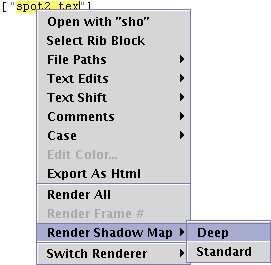Figures 6 The single parameter, shown below in red, tells the helper app ` Procedural "RunProgram" ["python canopy.py" "2.0"]` to generate 2000 small polygons. If the reader is using MacOSX, or Linux, the call to `Procedural` should specify the full path to the python interpreter ie. ` Procedural "RunProgram" ["/usr/bin/python canopy.py" "2.0"]`